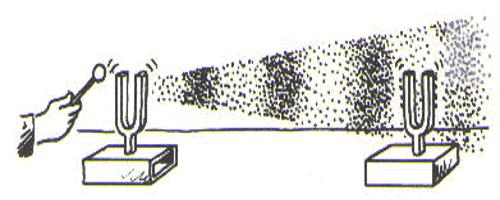# Resonance Explanation with Characteristics

Resonance Explanation with Characteristics

When the natural frequency of a body becomes equal to the frequency of forced vibration, then the body vibrates with maximum amplitude. This type of vibration is called resonance.

Explanation

(i) If a tuning fork is placed in air after vibration, a feeble sound is heard. But if this tuning fork is placed on a table, then strong sound is heard. In this case, due to the vibration of the tuning fork forced vibration is produced on the table and air around the table vibrates. Here amplitude of vibration increases, hence intensity of sound increases. In this way vibration is created [Figure].(ii) When soldiers move on marching over a hanging bridge keeping pace with one another a forced vibration is generated. As a result iron and other components of the bridge also vibrate. When the forced vibration and the vibrations of the components of the bridge become equal then the bridge vibrate with maximum amplitude. In this situation, the bridge may collapse. This is how resonance is created in a bridge.

Characteristics of resonance:

• When the natural time period of a body becomes equal to the time period of the force applied on it, then resonance is produced in the vibration of the body.
• All resonance vibrations are forced vibrations.
• Amplitude becomes maximum in resonance vibration.
• In resonance, within short tine of starting vibration, it becomes regular.
• Resonance structures should have the same number of electrons, do not add or subtract any electrons.